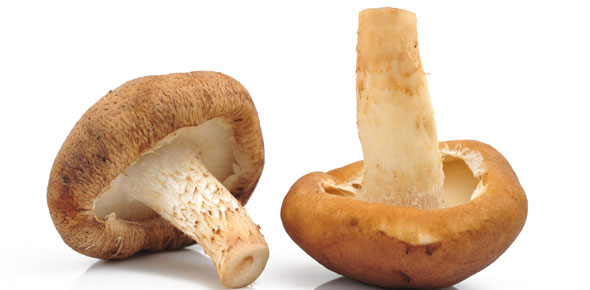A Lot Of, Many, Much

10 Questions | Total Attempts: 238SettingsA lot of, many, much

• 1.
There are __________ mushrooms.
• A.

A lot of

• B.

Many

• C.

Much

• 2.
There aren't _________ car parks.
• A.

Many

• B.

Much

• C.

A lot of

• 3.
There isn't __________ rice.
• A.

Much

• B.

Many

• C.

A lot of

• 4.
Are there ____________ supermarkets?
• A.

Many

• B.

Much

• C.

A lot of

• 5.
Is there __________ sugar?
• A.

Much

• B.

Many

• C.

A lot of

• 6.
There are _________ pet shops.
• A.

A lot of

• B.

Much

• C.

Many

• 7.
There is _______ noise.
• A.

A lot of

• B.

Much

• C.

Many

• 8.
Is there ______ butter?
• A.

Much

• B.

Many

• C.

A lot of

• 9.
There are ______ book shops.
• A.

A lot of

• B.

Much

• C.

Many

• 10.
There isn't ________ cream.
• A.

Much

• B.

Many

• C.

A lot of

Related TopicsBack to top